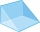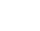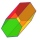# Volume + triangle - math problems

#### Number of problems found: 188

• Prism heightWhat is the prism's height with the base of a right triangle of 6 cm and 9 cm? The diaphragm is 10.8 cm long. The volume of the prism is 58 cm3. Calculate its surface.
• The cone - S,VCalculate the volume and surface area of the cone if its radius r = 6 cm and side s = 10 cm.
• Průměr kuželeVypočtěte povrch a objem rotačního kužele jehož průměr je 60mm a délka strany 3.4 cm.
• Iglu - cone tentThe cone-shaped tent is 3 m high, the diameter of its base is 3.2 m. a) The tent is made of two layers of material. How many m2 of fabric is needed for production (including flooring) if 20% needs to be added to the minimum amount due to cutting waste? b)
• Triangular pyramidCalculate the volume of a regular triangular pyramid with edge length a = 12cm and pyramid height v = 20cm.
• Pentagonal pyramidFind the volume and surface of a regular pentagonal pyramid with a base edge a = 12.8 cm and a height v = 32.1 cm.
• Find theFind the surface area of a regular quadrilateral pyramid which has a volume of 24 dm3 and a height of 45 cm.The height of a regular quadrilateral prism is v = 10 cm, the deviation of the body diagonal from the base is 60°. Determine the length of the base edges, the surface, and the volume of the prism.
• Surface of the coneCalculate the surface of the cone if its height is 8 cm and the volume is 301.44 cm3.
• CalculateCalculate the cone's surface and volume that results from the rotation of the right triangle ABC with the squares 6 cm and 9 cm long around the shorter squeegee.
• Cone - from volume surface areaThe volume of the rotating cone is 1,018.87 dm3, and its height is 120 cm. What is the surface area of the cone?
• Rotating coneFind the rotating cone's surface and volume if its side is 150 mm long and the circumference of the base is 43.96 cm.
• Cone - sideFind the cone's surface area and volume if its height is 125 mm and the side length is 17 cm.
• Digging a pitThe pit has the shape of a regular quadrilateral truncated pyramid. The edges of the bases are 14m and 10m long. The sidewalls form an angle of 135° with a smaller base. Determine how many m3 of soil were excavated when digging the pit?
• 9-gon pyramidCalculate the volume and the surface of a nine-sided pyramid, the base of which can be inscribed with a circle with radius ρ = 7.2 cm and whose side edge s = 10.9 cm.
• Maximum of volumeThe shell of the cone is formed by winding a circular section with a radius of 1. For what central angle of a given circular section will the volume of the resulting cone be maximum?
• Surface and volumeFind the surface and volume of the rotating cone if the circumference of its base is 62.8 m and the side is 25 m long.
• Sphere parts, segmentA sphere with a diameter of 20.6 cm, the cut is a circle with a diameter of 16.2 cm. .What are the volume of the segment and the surface of the segment?
• Hexagonal prismCalculate the volume and surface of a regular hexagonal prism with the edge of the base a = 6 cm with the corresponding height v1 = 5.2cm and the height of the prism h = 1 dm.What is the volume of a regular quadrilateral pyramid if its surface is 576 cm2 and the base edge is 16 cm?